# SAKURA-QiQi

## 实验2

x=list(range(10))

print('整数输出1：',end='')
for i in x:
print(i,end='')

print('\n整数输出2：',end='')
for i in x:
print(f'{i:0>2d}',end='-')

print('\n整数输出3：',end='')
for i in x[:-1]:
print(f'{i:02d}',end='-')
print(f'{x[-1]:02d}')

print('\n字符输出1：',end='')
y1=[str(i)for i in range(10)]
print('-'.join(y1))

print('字符输出：',end='')
y2=[str(i).zfill(2) for i in range(10)]
print('-'.join(y2))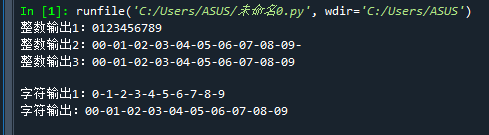name_list=['david bowie','louis armstrong','lenard cohen','bob dylan','cocteau twins']

for name in name_list:
print(name.title())

name_list_captilize=[name.title() for name in name_list]
print('\n'.join(name_list_captilize))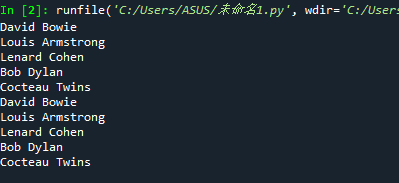name_list=['david bowie','louis armstrong','lenard cohen','bob dylan','cocteau twins']
name_list_captilize=[name.title() for name in name_list]
name_list_captilize.sort()

for i in range(5):
print(f'{i+1}.{name_list_captilize[i]}')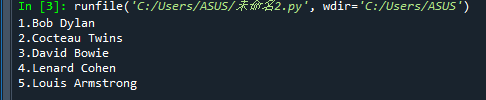zop='''
The Zen of Python, by Tim Peters
Beautiful is better than ugly.
Explicit is better than implicit.
Simple is better than complex.
Complex is better than complicated.
Flat is better than nested.
Sparse is better than dense.
Special cases aren't special enough to break the rules.
Although practicality beats purity.
Errors should never pass silently.
Unless explicitly silenced.
In the face of ambiguity, refuse the temptation to guess.
There should be one-- and preferably only one --obvious way to do it.
Although that way may not be obvious at first unless you're Dutch.
Now is better than never.
Although never is often better than *right* now.
If the implementation is hard to explain, it's a bad idea.
If the implementation is easy to explain, it may be a good idea.
Namespaces are one honking great idea -- let's do more of those!
'''

zop_lines=len(zop.splitlines())
zop_words=len(zop.split())
zop_char=len(zop)
zop_space=zop.count(' ')

print(f'行数：{zop_lines}')
print(f'单词数：{zop_words}')
print(f'字符数：{zop_char}')
print(f'空格数：{zop_space}')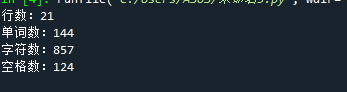book_list = [['静静的顿河','肖洛霍夫','金人', '人民文学出版社'],
['大地之上','罗欣顿.米斯特里','张亦琦', '天地出版社'],
['夜航西飞', '柏瑞尔.马卡姆', '陶立夏', '人民文学出版社'],
['来自民间的叛逆', '袁越', '','新星出版社'],
['科技与恶的距离', '珍妮.克里曼', ' 詹蕎語', '墨刻出版社'],
['灯塔','克里斯多夫.夏布特','吕俊君','北京联合出版公司'],
['小行星掉在下午','沈大成', '', '广西师范大学出版社']]
for i in range(7):
print(f'{i+1}.《{book_list[i]}》|{book_list[i]}|{book_list[i]}')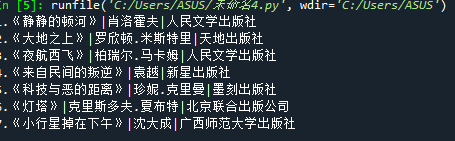data = ['99 81 75', '30 42 90 87', '69 50 96 77 89 93', '82 99 78 100']
r = 0
p = 0
for i in data:
x = i.split()
for y in x:
r += int(y)
p += 1
a = r / p
a1 = round(a, 2)
print(a1)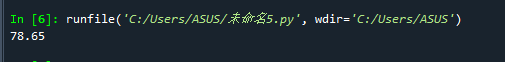words_sensitive_list = ['张三', 'V字仇杀队', '杀']
comments_list = ['张三因生命受到威胁正当防卫导致过失杀人，经辩护律师努力，张三不需负刑事责任。', '电影<V字仇杀队>从豆瓣下架了', '娱乐至死']
for y in comments_list:
for i in words_sensitive_list:
if i in y:
y = y.replace(i, '*' * len(i))
print(y)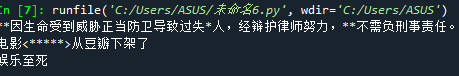posted on 2022-04-12 13:29  QiQi-sakura  阅读(10)  评论(2编辑  收藏  举报